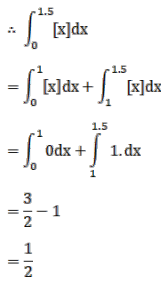# Solve this following

Question:

Mark $(\sqrt{ })$ against the correct answer in the following:

Let $[x]$ denote the greatest integer less than or equal to $x$.

Then,  $\int_{0}^{1.5}[x] d x=?$

A. $\frac{1}{2}$

B. $\frac{3}{2}$

C. 2

D. 3

Solution: